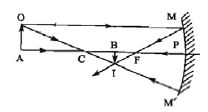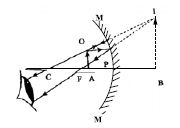# Explain Images formed in Concave Mirror

The position. size and nature of an image formed in a spherical mirror depend on the position of the object placed in front of the mirror. If there is a change in the position of the object, then a corresponding change in the position, size and nature of the image also takes place. Let, MPM’ be a concave mirror. Here, P is the pole, F is the principal focus and C is the centre of curvature of it. AO is the object situated perpendicularly on the principal axis in front of it.

If the object is placed anywhere between infinity and the principal focus, the image thus formed will always be real and inverted Again, in case of an object placed in between the principal focus and the pole, the image will be virtual and erect. The real and virtual images formed by a concave mirror are described below:

Real image: From the point O, a ray OM incident at the point M parallel to the principal axis and reflected through the principal focus along the path ME. Another ray OCIVI1 from the point 0 incident through the centre of curvature C on the mirror and reflects back along the same path. After reflection, the two rays meet at the point I actually. Therefore I am the real image of the point 0. From A. ray incident along the principal axis reflects back along the same path. So. the image of A will be formed on that line. Let us draw normal. IB, from the point I on the principal axis. Now. BI is the real image of the object AO (below figure). The nature of the image is real and inverted.Fig (1)

Virtual image: In figure 2, the object is situated between the pole and the principal focus. From the point 0, a ray incident parallel to the principal axis and reflected through the principal focus. Another ray incident on the mirror through the centre of curvature reflects back along the same path. After reflection, two rays become mutually diverging. If the two rays are extended backward, they appear to come from the point I. So, the point I am the virtual image of the point O. Normal B3 is drawn on the principal axis from the point I. therefore, BI is the virtual and erect image of the object. The position of the image so developed is behind the mirror, its nature is virtual and erect, and magnified i.e. larger than the size of the object.Fig (2)